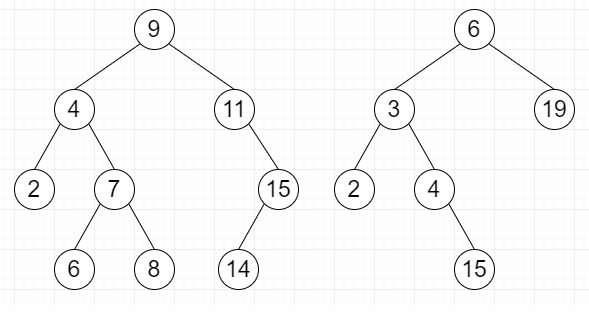# Find pairs with given sum such that pair elements lie in different BSTs in Python

Suppose we have two given Binary Search Trees and another sum is given; we have to find pairs with respect of given sum so that each pair elements must be in different BSTs.

So, if the input is like sum = 12then the output will be [(6, 6), (7, 5), (9, 3)]

To solve this, we will follow these steps −

• Define a function solve() . This will take trav1, trav2, Sum

• left := 0

• right := size of trav2 - 1

• res := a new list

• while left < size of trav1 and right >= 0, do

• if trav1[left] + trav2[right] is same as Sum, then

• insert (trav1[left],trav2[right]) at the end of res

• left := left + 1

• right := right - 1

• otherwise when (trav1[left] + trav2[right]) < Sum, then

• left := left + 1

• otherwise,

• right := right - 1

• return res

• From the main method do the following −

• trav1 := a new list, trav2 := a new list

• trav1 := in order traversal of tree1

• trav2 := in order traversal of tree2

• return solve(trav1, trav2, Sum)

## Example (Python)

Let us see the following implementation to get better understanding −

Live Demo

class ListNode:
def __init__(self, data):
self.data = data
self.left = None
self.right = None
def insert(root, key):
if root == None:
return ListNode(key)
if root.data > key:
root.left = insert(root.left, key)
else:
root.right = insert(root.right, key)
return root
def storeInorder(ptr, traversal):
if ptr == None:
return
storeInorder(ptr.left, traversal)
traversal.append(ptr.data)
storeInorder(ptr.right, traversal)
def solve(trav1, trav2, Sum):
left = 0
right = len(trav2) - 1
res = []
while left < len(trav1) and right >= 0:
if trav1[left] + trav2[right] == Sum:
res.append((trav1[left],trav2[right]))
left += 1
right -= 1
elif trav1[left] + trav2[right] < Sum:
left += 1
else:
right -= 1
return res
def get_pair_sum(root1, root2, Sum):
trav1 = []
trav2 = []
storeInorder(root1, trav1)
storeInorder(root2, trav2)
return solve(trav1, trav2, Sum)
root1 = None
for element in [9,11,4,7,2,6,15,14]:
root1 = insert(root1, element)
root2 = None
for element in [6,19,3,2,4,5]:
root2 = insert(root2, element)
Sum = 12
print(get_pair_sum(root1, root2, Sum))

## Input

[9,11,4,7,2,6,15,14], [6,19,3,2,4,5], 12

## Output

[(6, 6), (7, 5), (9, 3)]